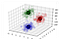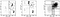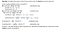# The Math Behind K-means Clustering

A step towards statistical modelling…K-means with k = 3

K-means Clustering algorithm is popular unsupervised machine learning algorithms, that solve the well-known clustering problem, with no pre-determined labels defined. It is often referred to as Lloyd’s algorithm.

The K-means clustering algorithm, tries to find out K number of centroids, and then allocates every data point to the nearest cluster. The ‘means’ in the K-means refers to averaging of the data; that is, finding the centroid. K refers to the total number of clusters to be defined in the entire dataset.

Algorithm:

• Step1: Randomly initialize the cluster centres of each cluster from the data points.

# The Math Behind PCA(Principal Component Analysis)

A step towards statistical modelling

Principal Component Analysis(PCA) is based on multivariate data analysis using projection methods.

PCA considers a Coordinate transformation (i.e. a rotation) from the arbitrary axes (or “features”) you started with to a set of axes ‘aligned with the data itself,’.

The central idea of PCA is in reducing the dimensionality of a data set consisting of a large number of interrelated variables while retaining variance of original dataset as much as possible.

# The Mathematics…

Let the original dataset be of k+1 dimensional, ignoring the class label dimension becomes k dimensional to reduce dimension further to d such that…

# The Math Behind Random Forest

A step towards Statistical analysis..fig(a): Decision Tree-1, fig(b): Decision Tree-2, fig(c): Forest

Ensemble means collection or group of things, Ensemble models in machine learning operate on a similar idea. Ensemble learning is a machine learning technique that combines several base models in order to produce one optimal predictive model.

Bagging or bootstrap aggregation is a technique for reducing the variance of an estimated prediction function. Bagging seems to work especially well for high-variance, low-bias procedures, such as trees.

Random forests is a substantial modification of bagging that builds a large collection of de-correlated trees, and then averages them.

ALGORITHM:

B: number of trees, T(b): b^th tree, C(b)…

# The Math Behind Decision Trees

Part1: A Statistical guide to Machine Learning

Decision tree is non-parametric , supervised learning algorithm used for Classification and Regression tasks. Decision tree classifier is based on the nested if-else classifier, it works through recursive partitioning of the training set in order to obtain subsets that are as pure as possible to a given target class. Each node of the tree is associated to a particular set of records 𝑇 that is splitted by a specific test on a feature.

There are Various algorithm that are used to generate decision tree from data, some are as following: (Classification and regression…

# The Math Behind SVM

part3: The Implementation

Let’s start, In this tutorial we would be building SVM classifier from scratch. We would be using python scripting language along with libraries for numeric calculations and visualization. This tutorial can be divided into 3 steps:

• Step1: Build dataset
• Step2: Train SVM, ie. defining fit method & Optimizing quadratic programming problem.
• Step3: Prediction

# 1. The Dataset

Let’s import libraries, and prepare linearly non-separable dataset for model

# 2. Train SVM

## a. Initialize SVM parameters:

C:= float, default=1.0 {Regularization parameter }

kernel{‘linear’, ‘poly’, ‘rbf’, ‘sigmoid’, ‘precomputed’}

degree: degree of polynomial kernel function

gamma{‘scale’, ‘auto’} or float, default=’scale’

tol :float, default=1e-3

max_iter: int default=-1

## b. Defining fit method of SVM

The training of SVMs…

# The Math Behind SVM

Part2: Lagrange multipliers & Optimizations

website: https://eliteaihub.com/

We can find the maximum or minimum of a multivariable function with some constraint using the Lagrange Multiplier. It’s important to understand Lagrange Multiplier to solve constraint optimisation problems, like we have in SVM.

Langrange Multipliers??

A contour plot is a way to visualise a three-dimensional function into two dimensions. Let’t say we want to find the maximum value of the multivariate function as shown below,

# The Math Behind SVM

Part 1: Implementing SVM from scratch

website: https://eliteaihub.com/

SVM or Support Vector Machine is a linear model for classification and regression problems. It can solve linear and non-linear problems and work well for many practical problems. The idea of SVM is simple: The algorithm creates a line or a hyperplane which separates the data into classes.

The goal of SVM is to identify an optimal separating hyperplane which maximises the margin between different classes of the training data.

We will be considering a linear classifier for a binary classification problem with labels y and features x.

# Human-Activity-Recognition using Transfer Learning and Sequential models

Domain Background:

website: https://eliteaihub.com/

Video Recognition ? Intuitively, videos are nothing but a running sequence of frames. So, we can say that a video is composed of multiple frames, stacked after one another. Now, an image (technically a digital image) is a 2-d array of pixels. These pixels may represent gray levels, intensity values etc., that make up the image. Also, if we are dealing with color images, there are three 2-dimensional arrays of these pixels, one for each of the 3 channels — Red(R), Green(G) and Blue(B).

Transfer Learning ? In simple terms, transfer learning is the process of…

# Addressing Viterbi Algorithm challenges

Part2: Viterbi decoding algorithm for POS tagging

website:https://eliteaihub.com/

In the last blog I discussed about how you can implement The Viterbi algorithm from scratch that can be used for decoding, finding the most likely tag sequence. Today we will identify some of the challenges and try to address them. Let’s go through the algorithm as well:viterbi algoritm

# 1. Underflow and Overflow

Except for rather short sequences in which n is relatively small, say, a couple hundred or so, the algorithm above will fail due to the fact that we are trying to represent numbers that are too small (or too large) for the computer to…

# Build Viterbi Decoding Model from Scratch

website: https://eliteaihub.com/

The Viterbi algorithm provides an efficient way of finding the most likely state sequence in the maximum a posteriori probability sense of a process assumed to be a finite-state discrete-time Markov process.

I highly recommend that you know the basics of Viterbi decoding algorithm before reading further. Check https://youtu.be/yGtC10JJNq8 for understanding basics of viterbi decoding algorithm.

Let’s get Started, You would be needing the following libraries installed before getting started. Import the libraries once the installation is done.

We would be using Treebank corpus and Universal Tagset. You can refer http://cse.iitkgp.ac.in/~sudeshna/courses/NLP19/Universal%20POS%20tags.html## EliteAI

Helping you build something Innovative…